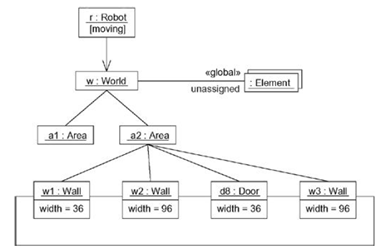UML Tutorial for Beginners
Blog containing resources for uml like lecture notes, lecture videos, lab manual, uml diagrams, objective bits, important questions and more.

10/08/2013 Categories: Structural Modeling.

Before learning about object diagrams in UML, have a look at class diagrams in UML.

An object diagram models the instances of classes in a class diagram. An object diagram contains objects and links connecting the related objects.

Object diagrams allow us to model the static design view or static process view. It involves modeling a snapshot of the system which contains objects (instances), their state and links between objects.

An object diagram represents the static part of an interaction containing the objects that communicate and the links between them without any messages.## Common Properties

An object diagram shares the same common properties like the rest of the diagrams, a name which identifies the diagram and graphical contents which are a projection into the model. What distinguish an object diagram from the rest of the diagrams are its contents.

## Contents

Object diagrams contain:

• Objects

Like all other diagrams, object diagrams may contain notes and constraints.

Object diagrams may also contain packages and subsystems which are used to group the related elements in the model together. Sometimes we can also include classes in the object diagrams. Generally, object diagrams are used to model the object structures.

## Common Modeling Techniques

### Modeling Object Structures

To model an object structure,

• First, identify the function or behavior or part of a system you want to model as collection of classes, interfaces and other things.
• For each function or mechanism identify the classes and interfaces that collaborate and also identify the relationships between them.
• Consider a scenario (context) that walks through this mechanism and freeze at a moment in time and identify the participating objects.
• Represent the state of objects by listing out the attributes and their values.
• Represent the links between objects which are instances of associations.### Forward and Reverse Engineering

Forward engineering a object diagram is theoretically possible but practically of limited value as the objects are created and destroyed dynamically at runtime, we cannot represent them statically.

To reverse engineer a object diagram,

1. Choose the target (context) you want to reverse engineer.
2. Use a tool to stop execution at a certain moment in time.
3. Identify the objects that collaborate with each other and represent them in an object diagram.
4. To understand their semantics, expose these object’s states.
5. Also identify the links between the objects to understand their semantics.